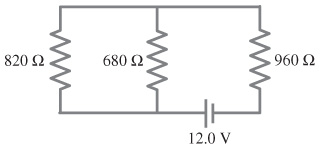+1 vote

editeda) Determine the equivalent resistance of the circuit shown in the figure.

b Determine the voltage across 820 Ω resistor.

c) Determine the voltage across 960 Ω resistor.

d) Determine the voltage across 680 Ω resistor.

+1 vote
by

Relative to the battery, the 820 and 680 resistors combine in parallel to form a smaller resistor so

(1/820) + (1/680) = (1/R1)

1/371.7333 ohms = 1/R1

R1 = 371.7 ohms

R1 and R2 are in series so

Rt = 371.7 + 960 = 1331.7 ohms = equivalent or total resistance

To find voltage across the resistors, you're first going to need the current in the circuit.

V = IR

I = Vt/Rt = 12 V/1331.7 ohms = 9.0110E-3 A

You can determine voltage using V = IR

so start with R1 (note that since the two resistors to the left are in parallel, they will share voltage V1 which = IR1)

V1 = (9.0110E-3 A) (371.7 ohms) = 3.3493 volts

from here you can say voltage on the 960 resistor is 12 - 3.3493 = 8.6507 volts but I'm going to calculate just to be safe

V2 = (9.0110E-3 A) (960 ohms) = 8.65056--close enough, lol

a) 1331.7 ohms

b) 3.3493 volts

c) 8.6507 volts just to stay consistent with the R1

d) 3.3493 volts

Lorem ipsum dolor sit amet, consectetur adipiscing elit, sed do eiusmod tempor incididunt ut labore et dolore magna aliqua. Ut enim ad minim veniam, quis nostrud exercitation ullamco laboris nisi ut aliquip ex ea commodo consequat. Duis aute irure dolor in reprehenderit in voluptate velit esse cillum dolore eu fugiat.
by
Mastering Physics Giancoli
Lorem ipsum dolor sit amet, consectetur adipiscing elit, sed do eiusmod tempor incididunt ut labore et dolore magna aliqua. Ut enim ad minim veniam, quis nostrud exercitation ullamco laboris nisi ut aliquip ex ea commodo consequat. Duis aute irure dolor in reprehenderit in voluptate velit esse cillum dolore eu fugiat.